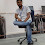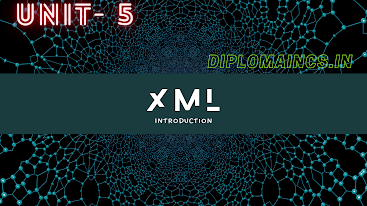## 2/15/20

### INTRODUCTION TO ALGORITHM DESIGN AND DATA STRUCTURES

UNIT: - 01

INTRODUCTION TO ALGORITHM DESIGN AND DATA STRUCTURES:

Design and analysis of algorithm: Algorithm definition

Comparison of algorithms

Analysis of Algorithm: Frequency count, Complexity measures in terms of time and space.

Design and analysis of algorithm: Algorithm definition: -

1.  A step-by-step procedure, which defines a set of instructions to be executed in a certain order to get the describe output, is termed as algorithm.

2.  A set of instructions or logic, which is written in order, to accomplish a certain predefined task is also called algorithm.

3.  Algorithm is not the complete code or programs, it is just the core solution or logic behind the program.

4.  Algorithm can be expressed either as an informal high level description as pseudo-code or using a flowchart.

There are some properties which must satisfy by the every algorithm: -

1.  INPUT: - Input must be 0 or more supplied externally to the algorithm.

2.    Output: - Output should be obtained 1 at least.

3.    Definiteness: - Each step of the algorithm should be well defined and clear.

4.    Finiteness: - The numbers of steps in any algorithm should have finite.

5.    Correctness: - The algorithm must generate a correct output at each or every step.

Comparison of algorithms

There are several types of Algorithm which are as follows: -

a.  Recursive algorithm

b.  Divide and conquer algorithm

c.   Dynamic programming algorithm

d.  Greedy algorithm

e.  Brute force algorithm

f.    Backtracking algorithm

1.  Recursive algorithm: -

a.  As the name suggests it’s a recursive method i.e. Algorithm that calls itself continuously until the problem is solved.

b.  It is one of the most interesting Algorithms as it calls itself.

c.   Tower of Hanoi and DFS (Depth First Search) of a graph, such types of problem can be solved easily using this algorithm.

2.  Divide and conquer algorithm: -

a.  These algorithm follows divide and conquer approach.

b.  The problems are break down into two sub-problems; each one is smaller than original.

c.   Again those two problems are divided into sub-problems.

d.  Solve the sub-problems recursively and then combine these solutions to get the solution of original problem.

e.  Merge sorting and quick sorting can be done using divide and conquer algorithm.

3.  Dynamic programming algorithm: -

a.  Dynamic programming algorithm solves complex problems by breaking it into multiple sub-problems.

b.   After breaking it, then solve each of them once and then stores for future use.

c.   We can apply this algorithm technique in while solving the problem of Fibonacci series.

4.  Greedy algorithm: -

a.  Greedy algorithms are used for solving expended/optimized problems.

b.  We find a locally optimum solution at the global level, in this algorithm.

c.   We can use this algorithm in Huffman coding and Dijkstra’s algorithm.

5.  Brute force algorithm: -

a.  Brute force algorithm is one of the simplest algorithms in concept.

b.  A brute force algorithm iterates all possible solutions which is search in one or more than one solution which may solve a function.

c.   Sequential search is preformed easily by using this function.

6.  Backtracking algorithm: -

a.  Technique to find a solution in an incremental approach is termed as Backtracking algorithm.

b.  Backtracking solves the problems recursively, and it tries to get to a solution to a problem by solving one piece of the problem at a time.

c.   In this case if one of the solutions fails, we will removed it and backtrack to find another solution.

d.  N queen’s problem can be easily solved, by using Backtracking algorithm.

Analysis of Algorithm: Frequency count, Complexity measures in terms of time and space: -

Algorithm analysis can be analyzed at two different stages first stage is before implementation and second stage is after implementation. Algorithm analysis is an important part of computational complicity theory which provides theoretical estimation to solve a specific computational problem. They are as follows: -

a.  A Priori Analysis: - The theoretical analysis of an algorithm can be termed as priori analysis. In this efficiency of an algorithm is measured by assuming that all other factors for example, processor’s speed.

b.  A Posterior Analysis: - The empirical analysis of an algorithm. In this algorithm is implemented using programming language.

ASYMPTOTIC NOTATION: -

When it comes to analysis the complexity of an algorithm in the terms of time and space, we cannot provide an exact or perfect time required and space required by an algorithm. Therefore we express it using some standard notations, which is also known as asymptotic notation.

It refers to computing the running time of any operation in mathematical unit of computation.

Time required by an algorithm falls under three types: -

a.  Worst case: -

When the time taken by any program execution is maximum, termed as worst case

b.  Best case: -

When the time taken by any program execution is minimum, termed as best case

c.   Average case: -

When the time taken by any program execution is average, termed as average case

There is some commonly used asymptotic notation which is used to calculate time complexity of an algorithm: -

a.    O notation (Big Oh notation)

b.   Ω notation (Omega notation)

c.     θ notation (Theta notation)

1.  Big Oh notation: -

1.  Big Oh notation O(n) is the formal way to express the upper bound of an algorithm’s running time.

2.  It can be also called as upper bound notation.

3.  It measures the worst case time complexity or the worst amount of time that can be taken by an algorithm.

4. For a function f(n)

` f(n) = O (g (n)) there exists c > 0 and n0 `
`such that f(n) ≤ c.g(n) for all n > n0. }`
` `

Example (linear equation): -

f(n)= 3n + 2, when n is at least 2

3n + 2 <= 3n + n <= 4n, so f(n) = O (n)

2.  Omega notation: -

1.  Omega notation is the formal way to express the lower bound of an algorithm’s running time.

2.  It can be also called as lower bound notation.

3.  It measures the best case time complexity or the best amount of time that can be taken by an algorithm.

4.  For a function f(n)

(f(n)) = Ω g(n) : there exists c > 0 and n0 such that g(n) ≤ c.f(n) for all n > n0. }

Example (linear equation): -

f(n)= 3n + 2 > 3n for all n, so f(n)= Ω(n). Also f(n) = 3n + 3 > 3n and so  (f(n)) = Ω g(n).

3.  Theta notation: -

1.    Theta notation is the formal way to express average bound (i.e. both lower case and upper case) of an algorithm’s running time.

2.    It can be also termed as average bound notation.

3.    It measures the average case time complexity or the average amount of time that can be taken by an algorithm.

4.    For a function f(n)

`          (f(n)) = θ g(n) if and only if g(n) =  Ο(f(n))`
` and g(n) = Ω(f(n)) for all n > n0. }`

(Below two points comes under complexity measure in terms of time and space)

Time complexity: -

1.  Algorithm which signifies the total time required by the program to run till its completion termed as time complexity.

2.  The time complexity algorithm is most commonly expressed by using big Oh notation (we have already know about Big Oh notation).

3.  The most commonly estimated by counting the number of elementary steps performed by any algorithm to finish execution, it is done by time complexity.

4.  Let’s take an example :-

Find square of a number a

for i= 1 to a

do a= a+a; /*when loops end “a” will hold its square*/

return a;

As we can see, loop will execute “a” numbers of time, so the time complexity of the above program is at least “a” number of times.

5.  Let’s take another example: -

Find square of a number b

return b*b;

In the above example time complexity will be constant, because it will not depend on the value of b. It will always give the result in one-step.

Space complexity: -

1.  As the name space means it is related to memory, in space complexity we will see how a solution of a problem required memory.

2.  Some memory is required to complete, when a solution to problem is written.

3.  Memory will be used for the solution of the program are following: -

a.  Variables

b.  Programs instructions

c.   Execution of the program

4.   Amount of memory which is used by the algorithm which includes the input values to the algorithm to execute and produce the results is called as space complexity.

5.  Space complexity is can be found by using below formula: -

Space complexity = Auxiliary space + Input space

Where,

Auxiliary space is extra space or temporary space used by the algorithm during execution.

6.  As we know about data types and size of it: -

Bool, char, takes 1 byte of memory space

int, short takes 2 bytes of memory space

folat, long takes 4 bytes of memory space

double, long long takes 8 bytes of memory space

7.  Let’s take an example and calculate space complexity: -

{

int z= a + b + c;

return (z);

}

Here a, b, c, and z all are integer types, so total memory required will be (4(4) + 4) = 20, 4 bytes will added for return value.

In the above example all the space requirement is fixed, therefore it is termed as Constant Space Complexity.

********************* THE END **********************

1.It's very nice sir
Easy to understand

1.Thank you..!!!!

2.It's easy language
Thank you ��

3.you are welcome..!!

2.1.you are welcome..!!

3.Tarif kru main uski jisne ye notes bnaya....really sir nice explanation...

1.ohh thank you so much..!!

4.Easy to understand 🙂

1.5.Very nice sir and tq

6.It's easy to understand. Thank you so much sir

Please do not enter any spam link in the comment box and use English and Hindi language for comment.

## Latest Update

### Key Components of XML